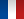• #### Number of hours

• Lectures 12.0
• Projects -
• Tutorials 12.0
• Internship -
• Laboratory works 20.0

ECTS 3.0

## Goal(s)

Provide a general overview of the properties of the main families of materials (in particular mechanical properties) and introduce tools for choosing materials.
Introduce the basics of the theory of the linear elasticity of solid bodies (stress, strain, generalised Hooke's law)
Introduce the use of a finite-element software for design purposes.

Responsible(s)

Barthelemy HARTHONG

## Content(s)

16h Initiation to materials (material properties with a focus on mechanical properties: elasticity, visco-elasticity, plasticity, fatigue, etc., normalised tests, main families of materials and typical values of properties, Ashby diagrams)
8h practicals (uniaxial pulling test)
8h basics of the theory of elasticity: stress tensor, strain tensor, generalised Hooke's law, equivalent stress (Mises)
8h practicals: use of finite-element software in the case of linear elasticity, mesh, boundary conditions

Prerequisites

Maths: differential and integral calculus in the case of multivariable functions, Taylor series, solid geometry, gradient, curl, divergence, divergence theorem, Matrix (tensor) calculus, basics of mechanics: 2nd and 3rd laws of Newton, concepts of force and moment

Test

CC 50% + CT 50%

Calendar

The course exists in the following branches:

• Curriculum - Master of Engineering GEE - Semester 7-8
see the course schedule for 2023-2024

Course language(s):